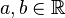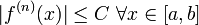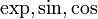# Difference between revisions of "Uniformly bounded derivatives implies globally analytic"

## Statement

### Global statement

Suppose$f$ is an infinitely differentiable function on$\R$ such that, for any fixed$a,b \in \R$, there is a constant$C$ (possibly dependent on$a,b$) such that for all nonnegative integers$n$, we have:$|f^{(n)}(x)| \le C \ \forall x \in [a,b]$

Then,$f$ is a globally analytic function: the Taylor series of$f$ about any point in$\R$ converges to$f$. In particular, the Taylor series of$f$ about 0 converges to$f$.

## Examples

The functions$\exp, \sin, \cos$ all fit this description.